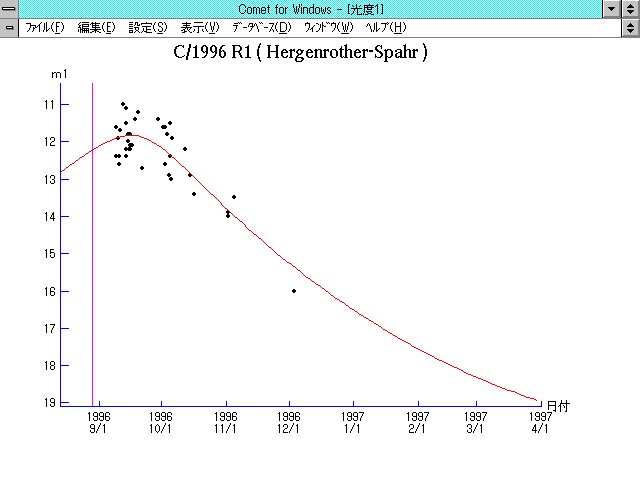# \$B%O!<%2%s%m!<%6!<(B-\$B%9%Q!<%kWB@1(B

C/1996 R1 ( Hergenrother-Spahr )###\$B%W%m%U%#!<%k(B

 \$BH/8+F|(B 1996\$BG/(B9\$B7n(B7\$BF|(B \$BH/8+8wEY(B 14\$BEy(B \$BH/8+ Carl W. Hergenrother, Timothy B. Spahr (Mount Bigelow)

###\$B50F;MWAG(B

```   The following improved orbital elements, by Kenji Muraoka, are
from 309 observations  1996 Sept. 8  to  Dec.  3, perturbations
by 9 Planets, Moon and 5 minor planets were taken into account.
The mean residual is +/- 0.60 arc seconds.

Epoch  =  1996 Aug. 25.0  TT       JDT = 2450320.5
T  =  1996 Aug. 28.73918       +/- 0.00107 (m.e.) TT
Peri. =  139.45613                +/- 0.00051
Node  =  149.74470                +/- 0.00054   (2000.0)
Incl. =  145.81455                +/- 0.00022
q  =    1.8992135              +/- 0.0000100 AU
e  =    0.9857025              +/- 0.0000840
1/a  =   +0.0075281              +/- 0.0000442 1/AU
( P  =   1531 years )
orig. 1/a  =   +0.0080976
fut.  1/a  =   +0.0076585
```

###\$B@1?^(B1995\$BG/(B12\$B7n(B 9\$BF|!A(B1996\$BG/(B 9\$B7n(B14\$BF|(B1996\$BG/(B 8\$B7n(B15\$BF|!A(B1996\$BG/(B11\$B7n(B 3\$BF|(B1996\$BG/(B10\$B7n(B24\$BF|!A(B1997\$BG/(B 8\$B7n(B20\$BF|(B

###\$B8wEYJQ2=(B

```        m1 = 6.2 + 5 log\$B&\$(B + 20.0 log r
```##### \$B50F;MWAG\$OB<2,7r<#;a\$N7W;;\$K\$h\$k\$b\$N\$G\$9!#(B \$B@1?^\$O(B StellaNavigator Ver.2.0 for Windows (\$B%"%9%H%m%"!<%D(B \$BJTCx(B / \$B%"%9%-!<=PHG6I4)(B) \$B\$G:n@.\$7\$?\$b\$N\$G\$9!#(B \$B8wEY%0%i%U\$O(BComet for Windows\$B\$G:n@.\$7\$?\$b\$N\$G\$9!#(B